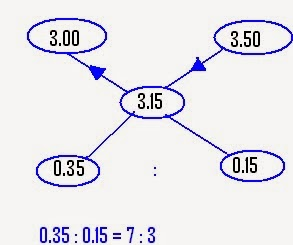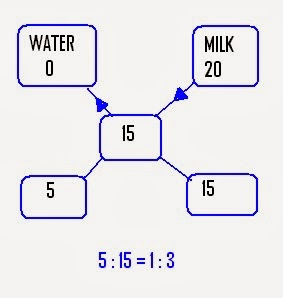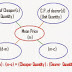Alligation means linking. Today I'm going to discuss alligation method with you all. This is one of an important chapters of Quantitative exams. Basically, alligation is a rule that enables us to find the ratio of the quantities of the ingredients at given prices and are mixed to produce a mixture of given price.

## Alligation

Alligation method is basically for two purpose:
• to find the mean value of the mixtures when prices and proportions of both the ingredients are given
• to find the proportion of an ingredients of mixture when prices of both ingredients and mixture are given

### Rule of Alligation

Let two ingredients are mixed, out of that one is dearer and other is cheaper comparatively.
Let Cost price (C.P.) of dearer one = d and
Cost price of cheaper one = c

Study the following figure, if you remember this, you can easily solve the questions related to this chapter. Always remember that you will always consider cost price, not the selling priceTherefore, (d-m) : (m-c) = (Cheaper Quantity) : (Dearer Quantity)
So, you can easily find the ratio of both the ingredients if prices of both the ingredients are given.

## Examples with Solution

Example1:  In what proportion rice @Rs3/kg be mixed with rice @ Rs 3.50/kg, so that mixture worth Rs. 3.15/kg?

Solution:  C.P. of cheaper rice = Rs 3/kg
C.P. of dearer rice = Rs 3.50/kg
Mean price i.e. price of mixture = Rs 3.15/kg

Applying the Alligation method,Therefore, Proportion in which both will be added is 7:3

Example2: A certain quantity of milk is mixed with 20 litres of water and the worth becomes Rs 15/litre. If price of pure milk is Rs 20/litre, then how much milk is there in the mixture?

Solution: Mixture = MILK + WATER
C.P. of pure milk = Rs 20/ltr

C.P. of water = Rs 0
Mean price i.e. price of mixture = Rs 15/ltr

Applying the Alligation method,Ratio of water to milk = 1:3
Therefore, quantity of milk in mixture = 3 times quantity of water
i.e. 3 times 20 = 60 litres

Example3: In what ratio must water be added to milk to gain 20% by selling the mixture at cost price?

Solution: In this ques, we will assume the cost price of milk so that we can simplify the problem.

Let assumed price (C.P.) of milk = Rs 10/litre

Then, according to ques, S.P. of milk = Rs 10/litre
Gain by selling mixture = 20%
Therefore selling price of mixture = Rs 120

C.P. = (100/120) times 10
C.P. of mixture = Rs(25)/3

By alligation method,
Ratio of water and milk = 1: 3

So, in this way we can calculate these types of problems. One more thing, if in case percentage is given instead of cost price, then also you can do the problem in the same way.

Hope you understand the topic. Will soon update more examples of related topic.
Also check:
Mixture or Alligation method of Quantitative AptitudeReviewed by Jasleen Behl on Thursday, January 30, 2014 Rating: 5